# The ICLIFETEST Procedure

### TEST Statement

• TEST variable </options> ;

In the preceding syntax, variable is a variable whose values determine the groups to be tested. The values for variable can be formatted or unformatted. If variable is a character or numeric variable, then the groups are defined by the unique values of the TEST variable. You can specify only one variable in the TEST statement.

When you are comparing more than two survival curves, a generalized log-rank test tells you whether the curves are significantly different from each other, but it does not identify which pairs of curves are different. A multiple-comparison adjustment of the p-values for the paired comparisons retains the same overall probability of a Type I error as the K-sample test. Two types of paired comparisons can be made: comparisons between all pairs of curves and comparisons between a control curve and all other curves. You use the DIFF= option to specify the comparison type, and you use the ADJUST= option to select a method of multiple-comparison adjustments.

Compared with the TEST statement in the LIFETEST procedure, the TEST statement in PROC ICLIFETEST is designed for comparing survival between predefined groups. Unlike the LIFETEST procedure, PROC ICLIFETEST does not support a similar test for detecting association with multiple covariates.

Table 62.2 summarizes the options available in the TEST statement.

Table 62.2: Options Available in the TEST Statement

Option

Description

Homogeneity Tests

Suppresses printing the test statistic and covariance matrix

Suppresses all tests

Names an output data set to contain the scores derived from the permutation form of the generalized log-rank test

Requests a trend test

Specifies tests that correspond to various weight functions

Multiple Comparisons

Requests a multiple-comparison adjustment

Specifies the type of differences to consider

You can specify the following options in the TEST statement after a slash ().

specifies the multiple-comparison method to use for adjusting the p-values of the paired tests. For mathematical details, see the section Multiple-Comparison Adjustments; also see Westfall et al. (1999). You can specify the following adjustment methods:

BONFERRONI
BON

applies the Bonferroni correction to the raw p-values.

DUNNETT

performs Dunnett’s two-tailed comparisons of the control group to all other groups. PROC ICLIFETEST uses the factor-analytic covariance approximation that is described in Hsu (1992) and identifies the adjustment in the results as "Dunnett-Hsu." ADJUST=DUNNETT is incompatible with DIFF= ALL.

SCHEFFE

performs Scheffé’s multiple-comparison adjustment.

SIDAK

applies the Šidák correction to the raw p-values.

SMM
GTE

performs the paired comparisons based on the studentized maximum modulus test.

TUKEY

performs the paired comparisons based on Tukey’s studentized range test. PROC ICLIFETEST uses the approximation that is described in Kramer (1956) and identifies the adjustment as "Tukey-Kramer" in the results. ADJUST=TUKEY is incompatible with DIFF= CONTROL.

SIMULATE <(simulate-options)>

computes the adjusted p-values from the simulated distribution of the maximum or maximum absolute value of a multivariate normal random vector. The simulation estimates q, the true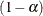quantile, where
is the value of the ALPHA= simulate-option.

The number of samples for the simulation adjustment is set so that the tail area for the simulated q is within a certain accuracy radius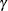of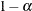, with an accuracy confidence of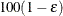%. In equation form,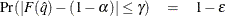where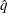is the simulated q and F is the true distribution function of the maximum; for more information, see Edwards and Berry (1987). By default,= 0.005 and= 0.01, so the tail area ofis within 0.005 of 0.95 with 99% confidence.

You can specify the following simulate-options:

ACC=value

specifies the target accuracy radiusof a% confidence interval for the true probability content of the estimatedquantile. By default, ACC=0.005.

ALPHA=value

specifies the valuefor estimating thequantile. The default value is the ALPHA= value in the PROC ICLIFETEST statement, or 0.05 if that option is not specified.

EPS=value

specifies the valuefor a% confidence interval for the true probability content of the estimatedquantile. The default value for the accuracy confidence is 99%, corresponding to EPS=0.01.

NSAMP=n

specifies the sample size for the simulation. By default, n is set based on the values of the target accuracy radiusand accuracy confidence% for an interval for the true probability content of the estimatedquantile. With the default values for,, and(0.005, 0.01, and 0.05, respectively), by default NSAMP=12604.

REPORT

specifies that a report of the simulation be displayed, including a listing of the parameters, such as,, and, in addition to an analysis of various methods of estimating or approximating the quantile.

SEED=number

specifies an integer used to start the pseudorandom number generator for the simulation. If you do not specify a seed, or if you specify a value less than or equal to 0, the seed is generated by default from reading the time of day from the computer’s clock.

DIFF=ALL | CONTROL<(’string’ <…, ’string’>)>

specifies which pairs of survival curves to consider for the multiple comparisons. You can specify the following values:

ALL

requests all paired comparisons.

CONTROL <(’string’ <…’string’>)>

requests comparisons of the control survival curve with all other survival curves. To specify the control curve, you specify the quoted strings of formatted values that represent the curve in parentheses. For example, if CELL=LARGE identifies the control group, you specify

   DIFF=CONTROL('large')


If more than one variable is used to identify the curves (for example, if CELL=LARGE and SEX=F represent the control), you specify

   DIFF=CONTROL('large' 'F')


The order of the quoted strings should correspond to the order of the TEST variable. If no string is specified as the control, the first group value is used.

By default, DIFF=ALL unless you specify ADJUST= DUNNETT, in which case DIFF=CONTROL.

NODETAIL

suppresses the display of the generalized log-rank statistics and the corresponding covariance matrices. If you specify the TREND option, the display of the scores for computing the trend test is suppressed.

NOTEST

suppresses the K-sample tests, stratified tests, and trend tests.

OUTSCORE=SAS-data-set
OUTSC=SAS-data-set

creates an output SAS data set to contain subject scores that are derived from a permutation form of the generalized log-rank statistic. For more information about the contents of the OUTSCORE= data set, see the section OUTSCORE= Data Set.

TREND

computes the trend test for the null hypothesis that the survival rates are the same for the groups versus an ordered alternative. If the TEST variable is numeric, the unformatted values of the variable are used as the scores; otherwise, the scores are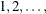in the order of the strata. For more information, see the section Trend Tests.

WEIGHT=test-request | (test-request <…test-request>)

requests weights to be applied to the generalized log-rank statistics to perform various tests. For more information about the various weight functions that the ICLIFETEST procedure supports, see the section Generalized Log-Rank Statistic. You can specify the following test-requests:

FAY

specifies the test that uses Fay’s weights (Fay 1999).

FINKELSTEIN

specifies the test that uses Finkelstein’s weights (Finkelstein 1986).

FLEMING(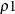,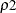)

specifies the family of tests in Harrington and Fleming (1982), whereandare nonnegative numbers. FLEMING(,) corresponds to the Fleming-Harrington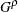family (Fleming and Harrington 1981) when=0. Alternatively, you can specify a test in this family as FLEMING(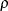) with one argument. When=0, the test becomes the log-rank test. When=1, the test should be very close to the Peto-Peto test.

NONE

suppresses all comparison tests. Specifying WEIGHT=NONE is equivalent to specifying NOTEST.

SUN

specifies the test that uses Sun’s weights (Sun 1996).